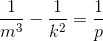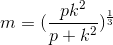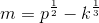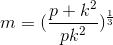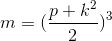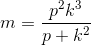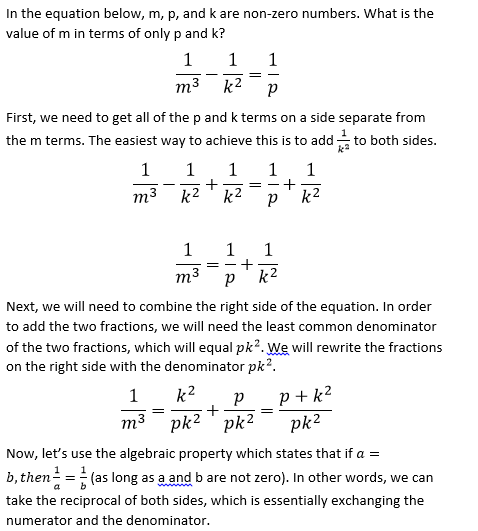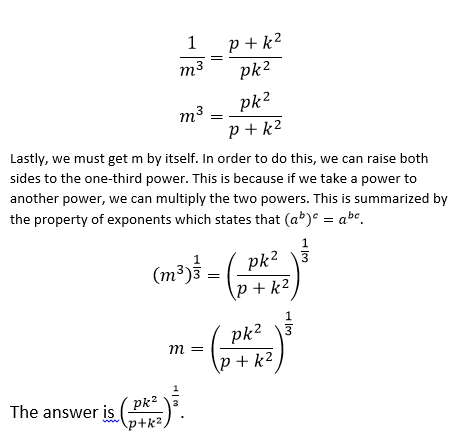# PSAT Math : How to find the solution to a rational equation with LCD

## Example Questions

### Example Question #3 : How To Find The Solution To A Rational Equation With Lcd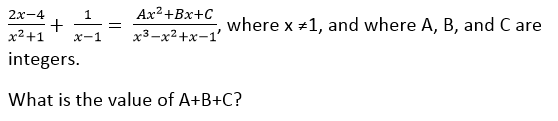–1

0

2

–2

1

2

Explanation: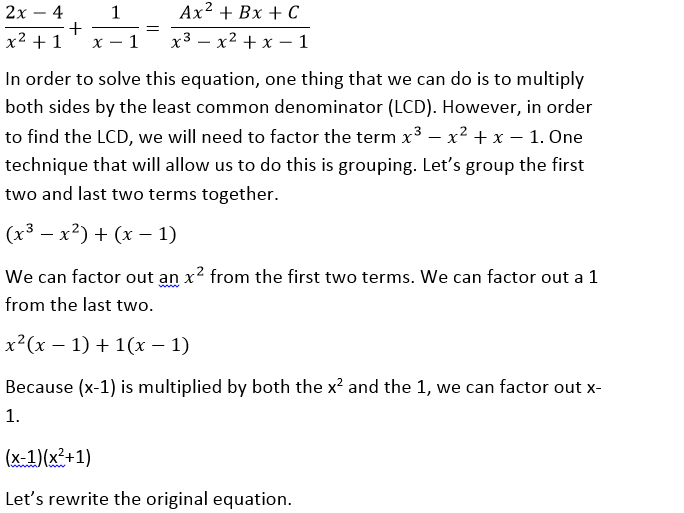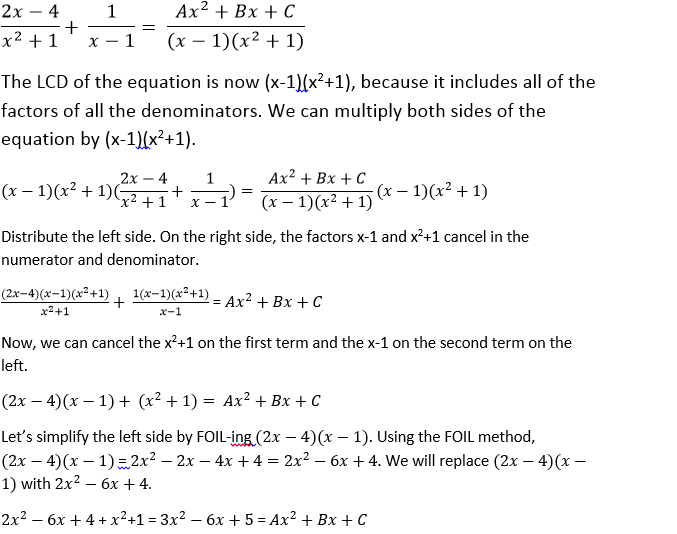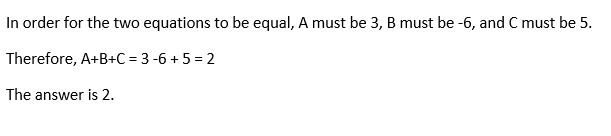### Example Question #51 : Algebra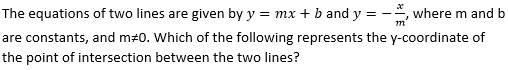b/(m– 1)

–bm/(m+ 1)

–b/(+ 1)

b/(m+ 1)

bm/(m+ 1)

b/(m+ 1)

Explanation: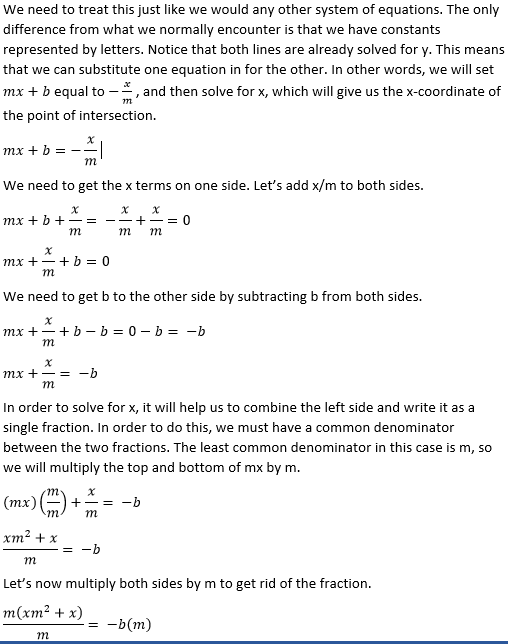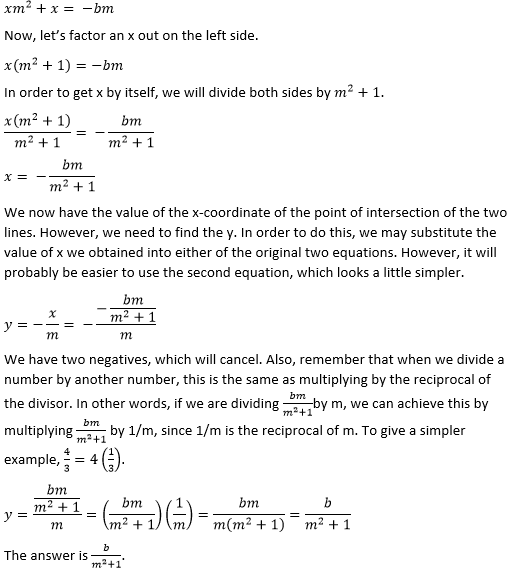### Example Question #5 : How To Find The Solution To A Rational Equation With Lcd

In the equation below,,, andare non-zero numbers. What is the value ofin terms ofand?